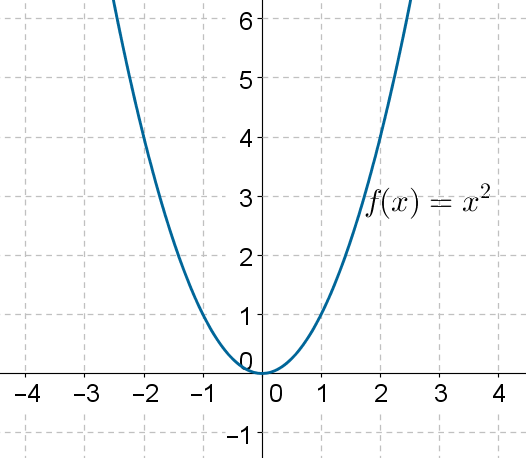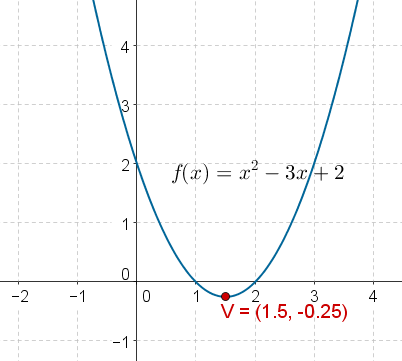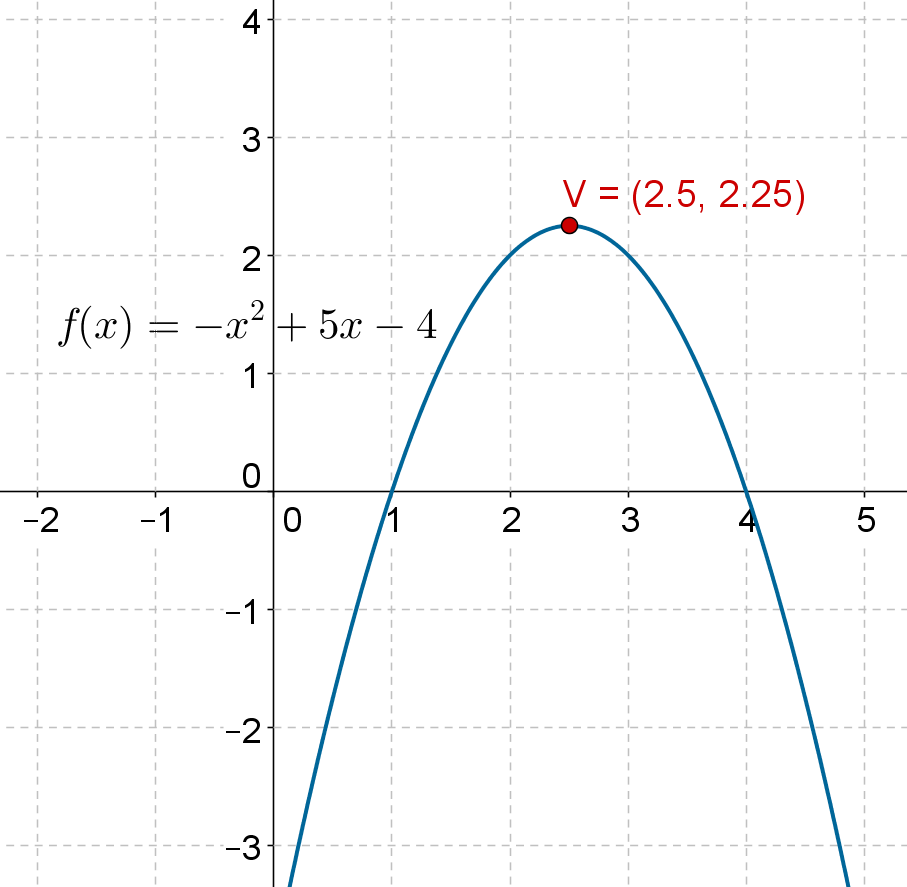Go back to  'Functions'

A quadratic function is a function of the form

$f\left( x \right) = a{x^2} + bx + c,\,\,\,a \ne 0$

The reason the coefficient needs to be non-zero is because if it is 0, then f will not have a quadratic term.

The simplest quadratic function is $$f\left( x \right) = {x^2}$$. The domain of this function is $$\mathbb{R}$$ . Also, because the output of f will take all non-negative values, the range of this function is $$\left[ {0,\infty } \right)$$.These facts are confirmed through the following graph of f:There is a point on the graph for every value of x which means that the domain is $$\mathbb{R}$$ ;and all y-values greater than or equal to 0 have corresponding points on the graph, which means that the range is $$\left[{0,\infty } \right)$$.

The following figure shows the graph of $$f\left( x \right) = {x^2} - 3x + 2$$:The domain of the function is clearly $$\mathbb{R}$$ . From the graph, we note that the lowermost point on the curve has a y-coordinate of $$- 0.25$$. Thus, the range of the function is $$\left[ { - 0.25,\infty } \right)$$.

The shape of the curve corresponding to any quadratic function is known as a parabola. Vertically, it extends infinitely in one direction. It may open upward or downward, depending upon the coefficient of the square term in the function definition. For both the quadratic functions above, their parabolas open upwards. The following figure shows the graph of $$f\left( x \right) = - {x^2} + 5x - 4$$:The parabola opens downwards, because the coefficient of $${x^2}$$ in the function definition is negative. Also, we see that the vertically, the curve does not go beyond $$y = 2.25$$, as the coordinates of the topmost point are $$\left({2.5,2.25} \right)$$. On the other hand, the curve extends indefinitely into the negative y-direction. Clearly, the range of the function is $$\left( { - \infty ,2.25} \right]$$. The domain is of course the set of all real numbers.

From the graph of a quadratic function, we can also determine its zeroes. For example, for the function above, the curve intersects the x-axis for $$x = 1,4$$. These are the two zeroes of the function.

We will soon discuss the plotting of quadratic functions in detail.

Functions
Functions
grade 10 | Questions Set 2
Functions
Functions
grade 10 | Questions Set 1